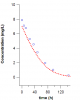# Fitting Experimental Data with a Differential EquationI would like to know how to define an ordinary differential equation(ODE) inside a curve fitting function. I'm a novice programmer in Igor, so I have been using the IntegrateODE command to check that I've written the correct ODE (e.g. Michaelis-Menten, see attached image ), but I still haven't been able to use it to fit any data set.

Function MM (pw,xx,yw,dydx)  // Michaelis-Menten relationship
//pw = Volume Distribution (Vd)
//pw = Maximal Elimination (Vmax)
//pw = Drug Concentration (Km)

Wave pw, yw, dydx
Variable xx

dydx = -pw*yw/(pw*(pw+yw))

return 0
End

Any ideas on how to use this function as part of a Fitting function of the form

Function myFitFunc(pw, yw, xw) : FitFunc
WAVE pw, yw, xw
yw = ....
End

The experimental data that I'm trying to fit is for a titration calorimetry experiment that has three fitting parameters. The binding constant (K), the binding enthalpy (H), and the molar equivalents (n). In this case, the signal measured is the differential heat (dq/dXt) after each injection and it can be expressed as:

d_q/d_Xt = d_XM/d_Xt * H * n

for the equilibrium reaction X + M ---> XM. In this case, the ODE is derived from the mass balance equations and the binding constant as follows:

Xt = X + XM
Mt = M + XM
K = XM/(X*M)

Mt*Xt - (Mt-Xt+1/K)*XM + XM^2 = 0

which gives the ODE

d_XM/d_Xt = (Mt -XM)/ (2*XM-Mt-Xt-1/K)

Function  OneSite_ODE(pw, xx, yw, dydx)
Wave pw
Variable xx
Wave yw
Wave dydx

//pw = Mt  total cocentration of  Macromolecule
//pw = Kb binding constant

dydx= (yw-pw)/(2*yw-pw-xx-(1/pw))

return 0
end

The OneSite_ODE has expected shape when Integrated an plotted against Xt. However, I was not able to fit my experimental data using the differential equation. This is the fitting function that I wrote, but it doesn't work.

Function OneSite(pw,wH,wX,wM )  : FitFunc

wave pw, wX, wM, wH
//pw = n
//pw = K
//pw = H
//pw = Lo , hold constant
//pw = Mo , hold constant
//pw = Vo , hold constant

Duplicate/O wX  XM
Make/O/D/n=2 pODE

pODE= pw
pODE= pw

XM=0
IntegrateODE OneSite_ODE, pODE , XM

wH = pw*pw*pw*(XM-wM)/(2*XM-wX-wM-(1/pw))

End

Any help would be greatly appreciated!I'm afraid that "it doesn't work" doesn't give me much to go on. Does it fail with a singular matrix error? Does it converge to a solution that doesn't have the correct shape? Does it fail with some other error message?

Your fitting function has the form of a multivariate fitting function (it has the coefficient wave, pw, the Y wave, wH, and two X waves, wX and wM) but the graph you show is a simple XY graph. That seems to imply that you have just one independent variable, not two. On the other hand, you didn't say what the graph shows, so maybe I've interpreted this incorrectly.

The fit coefficients pw isn't used. Your comments indicate that it will be held. If you truly have held that coefficient, it would be OK; if you forgot it would cause a singular matrix error.

Otherwise it seems like it should be OK.

John Weeks
WaveMetrics, Inc.
support@wavemetrics.com
Thanks for the reply John! I've had a little bit of progress this weeks with the differential equation approach to fit a curve. I've created some random data following the example in the manual for an exponential decay.
Make/N=500 xData, yData
xData = x/500 + gnoise(1/1000)
yData = 100 + 1000*exp(-.005*x) + gnoise(20)

Then, I defined the differential equation and the curve fitting function as follows:

Function ExpDecay (pw,xx,yw,dydx)
Wave pw, yw, dydx
Variable xx

dydx = -pw*yw

End

Function FitExpDecay(pw, yw, xw) : FitFunc
wave pw, yw,  xw

//pw= Y shift (y0)
//pw= Scalar (Ao)
//pw= Rate (a)

Duplicate/O/D yw yw1
Make/O/D/n=1 pODE
pODE=pw
yw1=pw
IntegrateODE /X=xw ExpDecay,  pODE, yw1
w= pw+yw1

End

I'm not sure if the parameter /X=xw is necessary in this case, but it gives better results. The values are closer to the ones generated with the CurveFit function. Here are the commands I used to generate the fits.

Make/O/D P2={10,900,0.1} //Initial guess
FuncFit/NTHR=0 FitExpDecay P2 YData /X=XData /D=fit_yData

CurveFit exp yData /X=xData /D
<pre><code class="language-igor">
That looks like you've made good progress! I have just a couple of comments on this:

1) The assignment in the fitting function must be an error in transferring it to the posting: it needs to be "yw" instead of "w"

2) You are correct that it is more accurate using /X=xw. That way the IntegrateODE solution points are exactly where you need them. Without it, you will get solution points at evenly-spaced intervals based on the wave scaling of the yw input wave. If the actual X values are not at those evenly-spaced X values, then Igor will do a linear interpolation, which won't be quite the same as the actual IntegrateODE solution.

3) At least for this problem, you don't need the intermediate wave yw1:
Function FitExpDecay(pw, yw, xw) : FitFunc
wave pw, yw,  xw

//pw= Y shift (y0)
//pw= Scalar (Ao)
//pw= Rate (a)

Make/O/D/n=1 pODE
pODE=pw
yw=pw
IntegrateODE /X=xw ExpDecay,  pODE, yw
yw += pw
End

That might a bit faster, saving the time required to make the intermediate wave.

John Weeks
WaveMetrics, Inc.
support@wavemetrics.com